# Behavior Study of Annealing Temperature on Microstructure and Wettability of Electrodeposited ZnO Thin Films for Microcapacitor Application

Behavior Study of Annealing Temperature on Microstructure and Wettability of Electrodeposited ZnO Thin Films for Microcapacitor Application

Moustafa Debbab Nassera Ghellai Omolayo M. Ikumapayi Noureddine Gabouze Giulio Lorenzini Chafiaa Yaddadene Malika Berouaken Younes Menni

Unite of Research on Materials and Renewable Energies – URMER, Department of Physics, Faculty of Sciences, Abou Bekr Belkaid University, Po. Box 119, 13000 Tlemcen, Algeria

Department of Mechanical and Mechatronics Engineering Afe Babalola University, 360101, Ado Ekiti, Nigeria

Division Couches Minces Surfaces Interfaces (CMSI), Centre de Recherche en Technologie des Semi-conducteurs pour l’Energétique (CRTSE), Algiers, Algeria

Department of Engineering and Architecture, University of Parma, Parco Area delle Scienze, 181/A, 43124 Parma, Italy

Department of Technology, University Center Salhi Ahmed Naama (Ctr. Univ. Naama), P.O. Box 66, Naama 45000, Algeria

Corresponding Author Email:
menniyounes.cfd@gmail.com
Page:
293-300
|
DOI:
https://doi.org/10.14447/jnmes.v25i4.a10
21 July 2022
|
Accepted:
23 October 2022
|
Published:
31 December 2022
| Citation

OPEN ACCESS

Abstract:

In this work, electrodeposition was used to produce thin zinc oxide layers on n-type silicon substrates. It has been investigated how annealing at $400^{\circ} \mathrm{C}$ affects the deposit's morphological and structural characteristics. SEM, X-ray diffraction, and contact angle measurements have all been used to study the morphology and structure of nonannealed and annealed $\mathrm{ZnO}$. Three intense peaks were visible in the $X R D$ patterns of non-annealed $Z n O$ thin films along the (100), (002) and (101) planes, however these peaks' intensities were different in the $X R D$ patterns of annealed $\mathrm{ZnO}$ layers. Furthermore, the $2 \theta$ diffraction angle shift can be seen in the $X R D$ patterns of annealed and unannealed $\mathrm{ZnO}$ layers. The contact angle measurements showed that the nonannealed ZnO layers are hydrophobic; however, at a temperature of $400^{\circ} \mathrm{C}$ for annealing, the ZnO layer surface becomes hydrophilic. Finally, $S E M$ data validate the $X R D$ and contact angle findings by showing how $Z n O$ structure changes from a sand rose shape to a granular structure. Additionally, the $\mathrm{ZnO} / n-$ Si micro-capacitor device has been developed and impedance spectroscopy (EIS) has been used to evaluate it. Finally, a microcapacitor made of $\mathrm{ZnO}$ and $n-S i$ showed a high specific capacitance of $128 \mathrm{mF} / \mathrm{g}$.

Keywords:

optoelectronics, sensing, energy storage, solar cells, microcapacitor, electrodes, superconductors, chemical vapor deposition, electrodeposition, ZnO elaborations

1. Introduction

Several studies on various semiconductor materials have been conducted over the past few decades, including zinc oxide $(Z n O)$, which has a wide band gap of $3.37 \mathrm{eV}$ and a high exciton binding energy ($60 \mathrm{meV}$) . $\mathrm{ZnO}$ has gained the consideration of numerous laboratories for its diverse properties such as structural and morphological, piezoelectric and pyroelectric and advantages: non-toxicity and abundance, etc [2-4]. $Z n O$ is a material that has found numerous uses in a variety of fields, including optoelectronics, sensing, energy storage or conversion as super capacitors, and solar cells, among others, as a result of its intriguing favorable features [5, 6]. Recently, several studies have been oriented to energy storage devices such as super capacitors using silicon material as a substrate combined with different materials especially carbon, transition metal oxides, polymer, etc [7, 8]. Thanks to its good scalability and easy integration with current silicon technologies, Silicon presents several advantages for its application in energy storage [9, 10]. Currently, a number of publications have been published about the remarkable pseudo-capacitive behaviors of transition metal oxides including $\mathrm{NiO}, \mathrm{V}_2 \mathrm{O}_5, \mathrm{RuO}_2, \mathrm{MnO}_2$ and $\mathrm{Co}_3 \mathrm{O}_4$ on silicon surfaces [11, 12]. It has been shown that these various Si/TMOs designs were revealed as good super-capacitor electrodes [9, 13, 14]. Due to its intriguing characteristics, including high capacity, natural abundance, and lastly low cost, $\mathrm{ZnO}$ has attracted the most attention among these different metal oxides as an electrode material for superconductors. Several methods of $\mathrm{ZnO}$ elaborations thin film were reported . However, these techniques have a number of drawbacks, including the requirement for bulky, expensive equipment and certain operating circumstances . The chemical approach, such as chemical vapor deposition (CVD) , sol-gel , spin coating, spray pyrolysis, and electrodeposition, involves creating the film through chemical reactions or molecular decompositions [17, 18]. The latter has been chosen in this work because of its several advantageous such as low cost, simple. In addition, the electrodeposition offers the possibilities to adjust the deposit properties such as the film thickness, shape, porosity, etc. improved interfacial bonding between the coating material and the substrate. With the use of an oxygen-rich media and a concentrated solution of $\mathrm{Zn}^{2+}+$ ions, thin films of $Z n O$ can be electrodeposited [19, 20].

In this work, a $\mathrm{ZnO}$ thin layer has been electrodeposited on n-type Si substrate and ZnO/n-type Si structure has been formed and studied. Calculations and evaluations have been done to determine how $400^{\circ} \mathrm{C}$ annealing temperature affects various $\mathrm{ZnO}$ properties, including $d_{h k l}$ (interplanar spacing), V (cell volume ), D (crystal size), $\Phi$ (dislocation density), and $\xi$ (micro - strain). XRD, SEM, $E D S$, and contact angle measurements were used to examine the structure and morphology of $Z n O$ thin films at the annealing temperature. The electrical properties of the $\mathrm{ZnO} / \mathrm{n}$ - Si diode construction have also been investigated. Through the use of the cyclic voltammetry method $(C V)$ and impedance spectroscopy (EIS), the device's capacitance behavior was examined. The $\mathrm{ZnO} / \mathrm{Si}-n$ device demonstrated a good specific capacitance, according to the results.

2. Experimental Methodology

2.1 Used chemicals

The substances listed in Table 1 were employed to create the solutions, whereas the chemicals products used to clean silicon are listed in Tables 2. The substrate is an $n-$ type silicon $(C Z)$ substrate with:

- Orientation: $(100) \pm 0.5^{\circ}$,

- Resistivity: $[0,007-0.013] \mathrm{cm}$, and

- Thickness: [275 - 325] $\mu \mathrm{m}$.

Table 1. Chemicals employed in the solutions' preparation

 Zinc Nitrate Hexahydrate $\mathrm{Zn}\left(\mathrm{NO}_3\right)_2-6 \mathrm{H}_2 \mathrm{O}$, SIGMA $-$ ALDRICH, $98 \%, 297.5105 \mathrm{~g} / \mathrm{mol}$ Potassium Nitrate $\mathrm{KNO}_3$, LABOSI, 99%, $101.11 \mathrm{~g} / \mathrm{mol}$ Sodium Sulfate $\mathrm{Na}_2 \mathrm{SO}_4, \mathrm{LABOSI}, 99 \%, 142.04 \mathrm{~g} / \mathrm{mol}$

Table 2. The cleaning chemicals items for silicon

 Trichlorethylene $\mathrm{C}_2 \mathrm{HCl}_3$, Prolabo, $99 \%, 131,39 \mathrm{~g} / \mathrm{mol}$ Acetone $\mathrm{C}_3 \mathrm{H}_6 \mathrm{O}$, Chemopharma, $99.78 \%, 50.08 \mathrm{~g} / \mathrm{mol}$ Ethanol $\mathrm{C}_2 \mathrm{H}_5 \mathrm{OH}$, Chemopharma, $95 \%, 46.07 \mathrm{~g} / \mathrm{mol}$ Acide Fluorohydrique HF, Chemopharma, $10 \%, 20.0063 \mathrm{~g} / \mathrm{mol}$

2.2 $\mathrm{ZnO}$ thin films electrodeposited

Through this experimental research, the chronoamperometry technique was used to electrochemically deposit zinc oxide layers on an $\mathrm{n}$ - type $\mathrm{Si}$ substrate. Prior to being cleaned in three ultrasonic baths, the silicon wafers are first sliced with a diamond tip into tiny silicon substrates measuring $1 \mathrm{~cm}$ by $1 \mathrm{~cm}$. The first one includes tricloro-amine that has been heated for 20 minutes at $60^{\circ} \mathrm{C}$. The substrates were then heated in acetone and ethanol at $55^{\circ} \mathrm{C}$ for 10 minutes each, followed by a DI water rinse and nitrogen gas flow drying of the wafers. Using a simple three-electrode setup and a straightforward electrochemical approach, the anodic electrodeposition of the layers of $\mathrm{ZnO}$ was carried out using $\mathrm{Si}-\mathrm{n}$ substrates as the working electrode and $\mathrm{Ag} / \mathrm{AgCl}$ as the reference electrode. The natural oxide layer on the silicon substrate was removed by immersing it in $10 \%$ hydrofluoric acid prior to each electrodeposition. The solution, which was made with deionized water (EDI), contains $6.10^{-3} \mathrm{M}$ of zinc nitrate hexahydrate $\mathrm{Zn}\left(\mathrm{NO}_3\right)_2-$ $6 \mathrm{H}_2 \mathrm{O}$, and $1.10^{-1} \mathrm{M}$ of potassium nitrate $\mathrm{KNO}_3$. During the electrodeposition, the temperature $(T)$ was maintained at $68^{\circ} \mathrm{C}$, and the deposition process took 30 minutes. An Autolab $128 \mathrm{~N}$ potentiostat-galvanostat and NOVA $1.8$ control software were utilized in all experiments. According to Peulon et al. , the method of increasing the local $\mathrm{pH}$ at the level of the working electrode was primarily used to carry out the electrochemical synthesis of $Z n O$. Several studies [19, 22, 23] claim that the reduction of nitrate ions into hydroxide ions, which then results in the precipitation of $\mathrm{Zn}(\mathrm{OH})_2$, is the first step in the electrochemical production mechanism of $\mathrm{ZnO}$. If the temperature is high enough; $68^{\circ} \mathrm{C}$ in the present investigation, Zinc II Oxide will develop from this hydroxide dihydrate, i.e., reaction 3. The equations 1, 2 and 3 can be used to summarize the order of $\mathrm{ZnO}$ deposition [24, 25].

$\mathrm{NO}_3^{-}+\mathrm{H}_2 \mathrm{O}+2 e^{-} \rightarrow \mathrm{NO}_2^{-}+2 \mathrm{OH}^{-}$          (1)

$\mathrm{Zn}^{2+} 2 \mathrm{OH}^{-} \rightarrow \mathrm{Zn}(\mathrm{OH})_2$           (2)

$\mathrm{Zn}(\mathrm{OH})_2 \rightarrow \mathrm{ZnO}+\mathrm{H}_2 \mathrm{O}$           (3)

Cyclic voltammetry has been used to determine the polarization voltage for the $\mathrm{ZnO}$ electrodeposition. Figure 1 displays cyclic voltammetry with an $\mathrm{Ag} / \mathrm{AgCl}$ electrode at a scan rate of $10 \mathrm{mV} \cdot \mathrm{S}^{-1}$ in the potential range of $-0.5$ to $1,6 \mathrm{~V}$. We see a peak around $-1.2 \mathrm{~V}$ during the return sweep, which is consistent with the production of $\mathrm{ZnO}$ . No peak has been seen during the reverse scan direction, indicating the absence of metallic zinc . According to numerous works [17, 24, 26], the applied voltage for the $Z n O$ plating should be around $-1.2 \mathrm{~V}$, according to the results.

## 1.png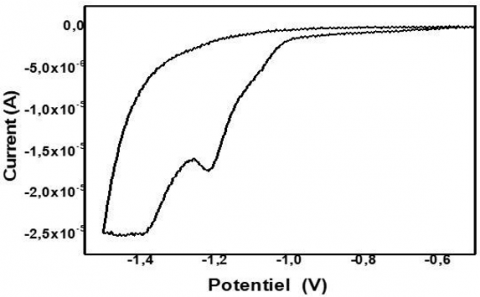Figure 1. Cyclic voltammogram produced at a rate of scan of $10 \mathrm{mV}^{-1}$ on an $\mathrm{n}$ - type silicon electrode (100) in an aqueous solution of $\left[\mathrm{Zn}^{2+}\right]=6.10^{-3} \mathrm{M}$

2.3 Characterisation of materials

An X-ray diffractometer (XRD, Ultima IV, Itm2036E302) with a radiation of $\lambda_{\text {CuKa }}=1.54059 \mathrm{~A}^{\circ}$, and a voltage generator of $40 \mathrm{kV}$ as well as a tube current of $40 \mathrm{~mA}$, were used to analyze the structural characteristics of the thin layer of $\mathrm{ZnO}$ with no annealing for $400^{\circ} \mathrm{C}$. They scanned the samples from 30 to 80 degrees at a slew rate of 5000 degrees per minute with a $0.05$ degree step. They used a DGIDROP (GBX) to measure the contact angle, depositing $3 \mu l$ drops of EDI deionized water on the substrate. An integrated digital camera that is managed by standard Visiodrop software is part of the system. Through the use of an electron microscope, the JEOL JSM-6360 LV, the morphologies of the acquired films have been examined.

3. Findings and Analyses

Figure 2 displays the $\mathrm{XRD}$ configurations of the $\mathrm{ZnO}$ layer that was deposited without annealing using the PDXL2 program that was included with the database (PDF 2 , release $2014,01-073-8765)$.

We can determine the Zn0 zincite structure ( hexagonal phase, space group P63mc (186)) from the identification bars overlaid on the peaks $31.7^{\circ}, 34.35^{\circ}$, $36.19^{\circ}, 47.55^{\circ}, 56.57^{\circ}, 62.74^{\circ}, 67.73^{\circ}, 67.83^{\circ}$, and $68.97^{\circ}$. The mesh parameters are $\mathrm{a}=\mathrm{b}=3.254167 \mathrm{~A}^{\circ}$ and $\mathrm{c}=$ $5.216132 \mathrm{~A}^{\circ}$. According to the acquired XRD patterns, the deposition of $\mathrm{ZnO}$ tends to have a preferred orientation (002) with the $c$ axis vertical to the substrate . These findings were supported by several works [20, 29, 30].

## 2.pngFigure 2. ZnO XRD structures, without annealing $T$

To achieve a good crystallinity following ZnO deposition, $\mathrm{T}$ management during annealing is crucial. Figure 3(a) compares the XRD structures of $\mathrm{ZnO}$ generated at a $400^{\circ} \mathrm{C}$ annealing $\mathrm{T}$ to the $\mathrm{ZnO}$ layer with no annealing. The Zn0 lattice layers of alignments (100), (002), and (101), respectively, produce 3 strong peaks in the XRD data with reflections from the Bragg angles at $\sim 31.7^{\circ}, 34.3^{\circ}$, and $36.2^{\circ}$, which correspond to the hexagonal type phase. The database (PDF $-2$, release $2014,01-073-8765)$ proved to be a good fit for the observed diffraction reflections. These findings further demonstrate that the formed ZnO layer is oriented in the direction of the $C(002)$ axis . When the Zn0 layer was annealed at 400 degrees Celsius, the peaks at $31.7,34.3$, and $36.2$ degrees became more pronounced, indicating good crystallinity and development of the $\mathrm{ZnO}$ thin layer. Figure 3(b) shows that the peak stations for the (100), (002) directions of the annealed ZnO layer were slightly displaced to better $2 \theta$ values, suggesting that greater crystallinity is attained and resulting in excellent accordance with that found by Shaban and Zayed .

## 3.png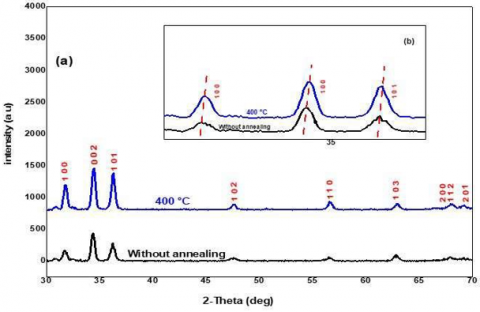Figure 3. (a) XRD models ZnO, (b) Comparison of peak angle values with and without, with/without annealing

The $\mathrm{ZnO}$ layer, which was electrochemically deposited in the absence and presence of annealing, respectively, is shown in Figures 4(a) and 4(b) together with SEM pictures and their morphology. The silicon substrate's surface is thickly and uniformly coated with a $Z n O$ deposit, as shown in Fig. 4(a-1).

## 4a.png(a)

## 4b.png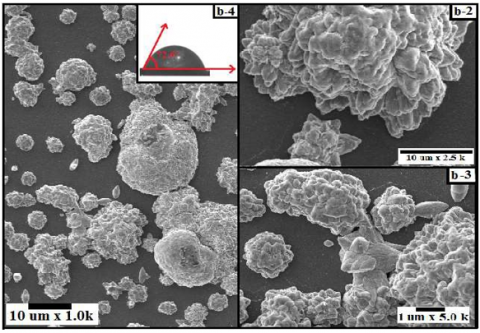(b)

Figure 4. (a) SEM images of $\mathrm{ZnO}$ thin films (a) with no annealing and (b) with $400^{\circ} \mathrm{C}$ annealing at different magnifications

These can be seen as rosette-tufts of sand that are perpendicular to the surface and contain hexagonal micropillars, as can be seen in the inset magnification of Figures 4 (a-2) and $4(\mathrm{a}-3)$, which shows how numerous writers came to this conclusion [22, 17]. Additionally, at a magnification of $1 \mu \mathrm{m}$, Figure $4(\mathrm{a}-2)$ reveals that the ends of the micro-pillars are tapered and have a mean diameter of $0.46 \mathrm{~m}$. The structure entirely transforms from a sandy pink model (see Figures 4(a1, 2, 3)) to a granular structure (see Figure 4(b-1,2,3) with the grains dispersed in various sizes and randomly aggregated in all orientations at the annealing temperature of $400^{\circ} \mathrm{C}$.

Measurements of the contact angle are widely used in order to examine the effects of surface treatments, porosity, and texturing . In our instance, we looked at how the $400^{\circ} \mathrm{C}$ annealing $\mathrm{T}$ affected the surface of the $\mathrm{ZnO}$. These measurements are made using a drop of $3 \mu \mathrm{l}$ of ultrapure ID (deionized water) at room $\mathrm{T}$. The $\mathrm{ZnO}$ layer was measured first with no being annealed, yielding an angle value of the contact of $122.1^{\circ}$ (see Figure 4(a-4)). When the deposit was annealed at $400^{\circ} \mathrm{C}$, the value of this same angle of the contact was $72.6^{\circ}$ (see Figure 4(b-4)). In the case with no annealing, a quantity greater than 90 indicates that the surface of the $\mathrm{ZnO}$ is hydrophobic .

Since DI is a polar substance that has a very high surface energy, this would mean that zinc oxide's surface is also polar but has a very small surface area. Energy coming into touch with the ZnO surface and causing the production of beads. After annealing at $400^{\circ} \mathrm{C}$, the contact value of the angle decreased from $122.1^{\circ}$ to $72.6^{\circ}$, revealing that the surface has become hydrophilic. The reduction in the surface energy and the conversion of $\mathrm{ZnO}$ bonds into polar bonds are further confirmed by this.

The crystal size value $(D)$ is calculated by :

$D=\frac{K \lambda}{\beta \cos \theta}$           (4)

With:

K: Scherer's constant (=0.9),

$\lambda:$ Wavelength $(0.15406 \mathrm{~nm})$,

$\theta:$ Peak position and the angle of Bragg diffraction in radians, and

$\beta:$ FWHM in radians (full-width-half-height).

The inter - planar spacing $\left(d_{h k l}\right)$ is computed by :

$d_{h k l}=\frac{n \lambda}{2 \sin \theta}$          (5)

With:

$n:$ Corresponds to the diffraction order $(=1)$,

$\lambda:$ X - ray wavelength $\left(1.5406 \mathrm{~A}^{\circ}\right)$, and

$\theta:$ Angle of diffraction in radians, and

The unit cell volume $(\mathrm{V})$ is estimated by :

$V=\sqrt{\frac{3}{2}} a^2 c$            (6)

Directly taken from the PDXL2 program, $a=b=$ $3.254167 \mathrm{~A}^{\circ}$, and $c=5.216132 \mathrm{~A}^{\circ}$. The dislocation density $\Phi$ is determined by :

$\Phi=\frac{1}{D^2}$          (7)

The micro-strain $(\xi)$ is reported as :

$\xi=\frac{\beta}{4 \tan \theta}$          (8)

Table 3 lists the $D, V, d_{h k l}, \Phi$, and $\xi$ values of the $Z n O$ layer both before and after annealing. Both with and with no annealing, the values for $d_{h k l}, V$, and $\Phi$ were observed. ZnO grains' crystal sizes grew from $18 \mathrm{~nm}$ with no annealing to $20.17 \mathrm{~nm}$ at a $400^{\circ} \mathrm{C}$ annealing $T$. On the other hand, the $\xi$ decreased when the $\mathrm{ZnO}$ layer was annealed at $400^{\circ} \mathrm{C}$, which means that the crystal lattice was harder and less detachable for the layer annealed at $400^{\circ} \mathrm{C}$ than for an unannealed layer. These results explain the previous difference in the $S E M$ image results (without annealing, see Figure 4(a-1); with annealing, see Figure 4(b-1). Which confirms that the reason for the difference in particle size (17.847 to $20.173 \mathrm{~nm}$ with and without annealing, respectively) is due to the change in their shape from the sand rose to the granular form. On the other hand, these results also lead to the change of the surface roughness, which changes the surface from hydrophobic to hydrophilic ( $122.1^{\circ}$ to $72.6^{\circ}$ with and without annealing, respectively).

Table 3. Values of $a, b, c, D, V, d_{h k l}, \Phi$, and $\xi$ for the $Z n O$ layer, before and after annealing

 Parameter Without annealing With annealing at $\mathbf{4 0 0}^{\circ} \mathrm{C}$ Crystallite size $(D), n m$ 17.847 20.173 Inter planar spacing $\left(d_{\mathrm{hkl}}\right)$, Å$^{\circ}$ 2.63 2.63 Lattice parameters $(a=b$, and $c),$ Å 3.25 and 5.21 3.25 and 5.21 Cell Volume $(V), A^{\circ 3}$ 47.836 47.836 Dislocation density $(\Phi)$, $n m^{-2}$ 0.144 0.144 Micro strain x 10-3 $(\xi)$ 6.76 5.86
4. Fabrication and Study of ZnO/Si-n Microcapacitor

In order to study the electrochemical performances of the prepared electrodes and to explore the advantages of the obtained morphologies and structure for the application of micocapacitors. Cyclic voltammetry $(C V)$ and spectroscopy (EIS) measurements were performed using a previously used three-electrode system with $1 \mathrm{M} \mathrm{Na}_2 \mathrm{SO}_4$ as the electrolyte solution. The working electrode contains the $\mathrm{ZnO} / \mathrm{Si}-n$ sample where the surface of the $Z n O$ is in direct contact with the electrolyte, the backside is completely covered with an insulating glue.

The $C V$ is an interesting method to assess the capacitive behavior of electrode materials. It is a technique widely used to obtain a qualitative knowledge of electrochemical reactions . The $\mathrm{CV}$ curves of the $\mathrm{ZnO} / \mathrm{Si}-n$ electrode without and annealed at $400^{\circ} \mathrm{C}$, at different scanning speeds $(10,20,50$, $100,200 \mathrm{mv} / \mathrm{s}$ ), immersed in the $1 \mathrm{MNa}_2 \mathrm{SO}_4$ electrolyte in the voltage range from $-0.8 \mathrm{~V}$ to $0.2 \mathrm{~V}$ are shown in Figures 5 (a) and 5(b), respectively. The same behavior is observed for both electrodes (with and without annealing). Also, it shows that at a constant bias potential, the current increases with increasing sweep rate without any distortion of the $C V$ shape at high sweep rate, which indicates an ideal capacitive property . Moreover, the preservation of the shape of the $C V$ curves at higher sweep speeds is a good indication for a better reversibility as suggested by several authors [38, 39].

## 5a.png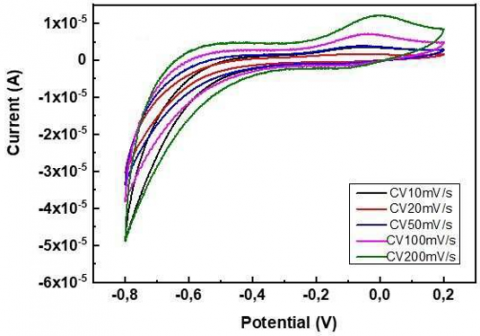(a)

## 5b.png(b)

Figure 5. Cyclic voltammetry curves of $\mathrm{ZnO} / \mathrm{Si}-n$ electrode: (a) without and (b) with annealing at $400^{\circ} \mathrm{C}$, for various scan rates $(10,20,50,100$, and $200 \mathrm{mV} / \mathrm{s})$, in $1 \mathrm{M}$ $\mathrm{Na}_2 \mathrm{SO}_4$ electrolyte

The calculation of the specific capacitance $C p$ in $(\mathrm{mF} / \mathrm{g})$ of the $\mathrm{ZnO}$ layer was made following the relation (9) . Where $\int I d v$ implies the area of the $C V$ curve, when the $Z n O$ surface capacitor is in contact with the charged and discharged electrolyte, $\mathrm{m}$ designates the mass in $(g)$ of the active electrode, $v$ specifies the scanning speeds in $(V / s)$, and $\Delta v$ is the potential window in $(V)$.

$C_p=\frac{\int_{V_1}^{V_2} I \partial V}{2 \cdot v \cdot m \cdot \Delta v}$          (9)

The specific capacities of the $\mathrm{ZnO} / \mathrm{Si}-n$ structure without annealing and with annealing at $400^{\circ} \mathrm{C}$ as a function of the scanning speed are represented in Figure 6 . It shows, foremost, that the two curves present a similar shape and which decreases with the increase in the scanning speed . At low sweep speeds $(10 \mathrm{mV} / \mathrm{s})$, the specific capacitance has large values of $218 \mathrm{mF} / \mathrm{g}$ and $186 \mathrm{mF} / \mathrm{g}$, the specific capacitance decreases to about $30-37 \mathrm{mF} / \mathrm{g}$ as the speed increases to $200 \mathrm{mV} / \mathrm{s}$ ), respectively for the $\mathrm{ZnO}$ layer without annealing and with at $400^{\circ} \mathrm{C}$. The accumulation of a high number of charges on the surface of the electrode, which leads to a high capacitance value, explains the behavior observed at low sweep speed. While at higher slew rates the charge mobility per unit time increases resulting in less charge accumulations at the electrode surface, which lower the capacitance value , it can be noted that the annealing of the $\mathrm{ZnO} / \mathrm{Si}-n$ slightly increases the specific capacity except at the sweep speed $10 \mathrm{mV} / \mathrm{s}$.

## 6.png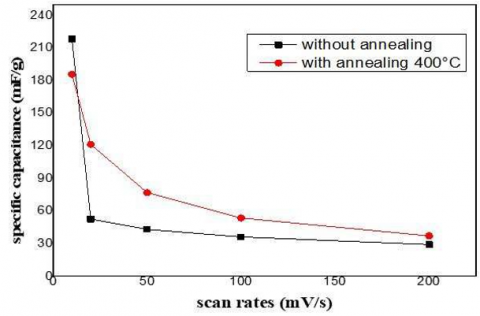Figure 6. Specific capacitance curves of $\mathrm{ZnO} / \mathrm{Si}-n$ as a function of scan rate, without and with annealing at $400^{\circ} \mathrm{C}$, for various scan rates $(10,20,50,100$, and $200 \mathrm{mV} / \mathrm{s})$, in $1 \mathrm{M} \mathrm{Na}_2 \mathrm{SO}_4$ electrolyte

The study of the charge transfer properties and the ionic diffusion of the $Z n O$ thin layer as a working electrode was carried out by electrochemical impedance spectroscopy (EIS) measurements under open circuit potential $(\mathrm{OCP})$ in $\mathrm{Na}_2 \mathrm{SO}_4$ electrolyte with a frequency range from $100 \mathrm{kHz}$ to $10 \mathrm{mHz}$. The Nyquist diagram, $Z^{\prime \prime}=f\left(Z^{\prime}\right)$ of the $Z n O / S i-n$ structure with annealing and without annealing and the fitted curve using a Randles circuit are represented in Figures 7(a) and $7(\mathrm{~b})$, respectively. The two figures exhibited a semicircle corresponding to charge transfer resistances Rct and a straight line, inclined with respect to the $Z^{\prime}$ axis, corresponds to the electrode effect (Warburg impedance). According to the fitted curves obtained by using the Randles equivalent circuits, the series resistance $R s$ determined from the intercept of the Nyquist diagram with $Z$ 'axis in the high frequency region are found of about $0(\Omega)$ for the two structures with and without annealing. However, the charge transfer resistance of the annealed structure is found higher $(450 \Omega)$ than the nonannealed structure $(126 \Omega)$ indicated a better charge transfer from the solution to the non-annealed structure surface.

5. Conclusions

On an $\mathrm{n}$-type silicon substrate, $\mathrm{ZnO}$ thin films have been successfully created using the electrodeposition technique. It has been designed and investigated to have a $\mathrm{ZnO} / \mathrm{Si}-n$ structure. It is also investigated how the annealing temperature affects the morphology and structure of $\mathrm{ZnO}$. The findings indicated that annealing at $400^{\circ} \mathrm{C}$ increases crystallinity, resulting in a maximum grain size of $20.17 \mathrm{~nm}$ for $\mathrm{ZnO}$.

## 7a.png(a)

## 7b.png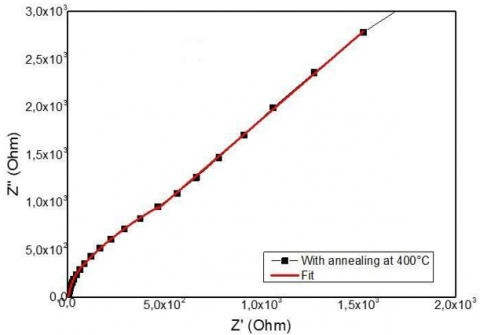(b)

Figure 7. Impedance spectroscopy (EIS) measurements under open circuit potential $(O C P)$ in $1 \mathrm{M} \mathrm{Na}_2 \mathrm{SO}_4$ electrolyte, and the Nyquist plot of the $\mathrm{ZnO} / \mathrm{Si}-n$ electrode (a) without and (b) with annealing at $400^{\circ} \mathrm{C}$, for frequencies from $100 \mathrm{kHz}$ to $10 \mathrm{mHz}$

Our research has also revealed that the micro-strain is decreased at this annealing $\mathrm{T}$, and that the interplanar spacing, dislocation density, and cell volume are comparable to those of unannealed samples. Following annealing at $400^{\circ} \mathrm{C}$, the $\mathrm{ZnO}$ layer underwent a structural transition from hydrophobic to hydrophilic, as seen by the contact angle measurements. It was concluded from SEM images that the annealing $T$ entirely alters the structure and morphology of the zincoxide films that were electrochemically produced. With no annealing, the $\mathrm{ZnO}$ layer displayed a sand rose type structure, which turns granular at $400^{\circ} \mathrm{C}$. The $\mathrm{ZnO} / \mathrm{Si}-n$ device's electrical properties, as determined by cyclic voltammetry $(\mathrm{CV})$ and impedance spectroscopy (EIS), showed high specific capacitances of 218 and $185 \mathrm{mF} / \mathrm{g}$ for the two structures with and without annealing, respectively. Additionally, the specific capacitance for the two structures decreases to 30 and $37 \mathrm{mF} / \mathrm{g}$ at low and high scanning rates, respectively. We also discovered excellent electrical properties for the $\mathrm{ZnO} / \mathrm{Si}-n$ structure without annealing, such as a very low series resistance (almost 0 ) and a low charge transfer resistance of $126 \Omega$.

References

 Akir, S., Barras, A., Coffinier, Y., Bououdina, M., Boukherroub, R., & Omrani, A. D. (2016). Eco-friendly synthesis of ZnO nanoparticles with different morphologies and their visible light photocatalytic performance for the degradation of Rhodamine B. Ceramics International, 42(8), 10259-10265.

 Barka-Bouaifel, F., Sieber, B., Bezzi, N., Benner, J., Roussel, P., Boussekey, L., ... & Boukherroub, R. (2011). Synthesis and photocatalytic activity of iodine-doped ZnO nanoflowers. Journal of Materials Chemistry, 21(29), 10982-10989.

 Taabouche, A., Bouabellou, A., Kermiche, F., Hanini, F., Sedrati, C., Bouachiba, Y., & Benazzouz, C. (2016). Preparation and characterization of Al-doped ZnO piezoelectric thin films grown by pulsed laser deposition. Ceramics international, 42(6), 6701-6706.

 Bilgin, V., Sarica, E., Demirselcuk, B., & Turkyilmaz, S. (2018). Iron doped ZnO thin films deposited by ultrasonic spray pyrolysis: structural, morphological, optical, electrical and magnetic investigations. Journal of Materials Science: Materials in Electronics, 29(20), 17542-17551.

 Rahal, B., Boudine, B., Souami, N., Siad, M., Sebais, M., Halimi, O., & Guerbous, L. (2020). Behavior study of the nanostructured zn1-xcdxo (0≤ x≤ 0.1) semiconductor thin films deposited onto silicon substrate by dip-coating method. Silicon, 12(12), 2967-2976.

 Sun, L., He, H., Liu, C., Lu, Y., & Ye, Z. (2011). Controllable growth and optical properties of ZnO nanostructures on Si nanowire arrays. CrystEngComm, 13(7), 2439-2444.

 Freyman, C. A., & Chung, Y. W. (2008). Synthesis and characterization of hardness-enhanced multilayer oxide films for high-temperature applications. Surface and Coatings Technology, 202(19), 4702-4708.

 Aradilla, D., Bidan, G., Gentile, P., Weathers, P., Thissandier, F., Ruiz, V., ... & Sadki, S. (2014). Novel hybrid micro-supercapacitor based on conducting polymer coated silicon nanowires for electrochemical energy storage. Rsc Advances, 4(50), 26462-26467.

 Thissandier, F., Gentile, P., Pauc, N., Brousse, T., Bidan, G., & Sadki, S. (2014). Tuning silicon nanowires doping level and morphology for highly efficient micro- supercapacitors. Nano Energy, 5, 20-27.

 Berton, N., Brachet, M., Thissandier, F., Le Bideau, J., Gentile, P., Bidan, G., ... & Sadki, S. (2014). Wide- voltage-window silicon nanowire electrodes for micro- supercapacitors via electrochemical surface oxidation in ionic liquid electrolyte. Electrochemistry Communications, 41, 31-34.

 Bencheikh, Y., Harnois, M., Jijie, R., Addad, A., Roussel, P., Szunerits, S., ... & Boukherroub, R. (2019). High performance silicon nanowires/ruthenium nanoparticles micro-supercapacitors. Electrochimica Acta, 311, 150- 159.

 Bao, J., Zhang, X., Bai, L., Bai, W., Zhou, M., Xie, J., ... & Xie, Y. (2014). All-solid-state flexible thin-film supercapacitors with high electrochemical performance based on a two-dimensional V 2 O 5· H 2 O/graphene composite. Journal of Materials Chemistry A, 2(28), 10876-10881.

 Thissandier, F., Dupré, L., Gentile, P., Brousse, T., Bidan, G., Buttard, D., & Sadki, S. (2014). Ultra-dense and highly doped SiNWs for micro-supercapacitors electrodes. Electrochimica Acta, 117, 159-163.

 Desplobain, S., Gautier, G., Semai, J., Ventura, L., & Roy, M. (2007). Investigations on porous silicon as electrode material in electrochemical capacitors. physica status solidi c, 4(6), 2180-2184.

 Huang, Z. H., Song, Y., Feng, D. Y., Sun, Z., Sun, X., & Liu, X. X. (2018). High mass loading MnO2 with hierarchical nanostructures for supercapacitors. ACS nano, 12(4), 3557-3567.

 Tynell, T., & Karppinen, M. (2014). Atomic layer deposition of ZnO: a review. Semiconductor Science and Technology, 29(4), 043001.

 Ahmed, N. A., Fortas, G., Hammache, H., Sam, S., Keffous, A., Manseri, A., ... & Gabouze, N. (2010). Structural and morphological study of ZnO thin films electrodeposited on n-type silicon. Applied surface science, 256(24), 7442-7445.

 Peulon, S., & Lincot, D. (1996). Cathodic electrodeposition from aqueous solution of dense or open‐ structured zinc oxide films. Advanced Materials, 8(2), 166-170.

 Abdelfatah, M., Salah, H. Y., El-Henawey, M. I., Oraby, A. H., El-Shaer, A., & Ismail, W. (2021). Insight into Co concentrations effect on the structural, optical, and photoelectrochemical properties of ZnO rod arrays for optoelectronic applications. Journal of Alloys and Compounds, 873, 159875.

 Arslan, A., Hür, E., Ilican, S., Caglar, Y., & Caglar, M. (2014). Controlled growth of c-axis oriented ZnO nanorod array films by electrodeposition method and characterization. Spectrochimica Acta Part A: Molecular and Biomolecular Spectroscopy, 128, 716-723.

 Peulon, S., & Lincot, D. (1998). Mechanistic study of cathodic electrodeposition of zinc oxide and zinc hydroxychloride films from oxygenated aqueous zinc chloride solutions. Journal of the Electrochemical Society, 145(3), 864.

 Yang, S., Sha, S., Lu, H., Wu, J., Ma, J., Wang, D., & Sheng, Z. (2020). Electrodeposition of hierarchical zinc oxide nanostructures on metal meshes as photoanodes for flexible dye-sensitized solar cells. Colloids and Surfaces A: Physicochemical and Engineering Aspects, 594, 124665.

 Kumar, K. D. A., Ganesh, V., Valanarasu, S., Shkir, M., Kulandaisamy, I., Kathalingam, A., & AlFaify, S. (2018). Effect of solvent on the key properties of Al doped ZnO films prepared by  nebulized  spray  pyrolysis technique. Materials Chemistry and Physics, 212, 167- 174.

 Mahalingam, T., John, V. S., Raja, M., Su, Y. K., & Sebastian, P. J. (2005). Electrodeposition and characterization of transparent ZnO thin films. Solar energy materials and solar cells, 88(2), 227-235.

 Kara, R., Mentar, L., & Azizi, A. (2020). Synthesis and characterization of Mg-doped ZnO thin-films electrochemically grown on FTO substrates for optoelectronic applications. RSC advances, 10(66), 40467-40479.

 Baka, O., Azizi, A., Velumani, S., Schmerber, G., & Dinia, A. (2014). Effect of Al concentrations on the electrodeposition and properties of transparent Al-doped ZnO thin films. Journal of Materials Science: Materials in Electronics, 25(4), 1761-1769.

 Kemell, M., Dartigues, F., Ritala, M., & Leskelä, M. (2003). Electrochemical preparation of In and Al doped ZnO thin films for CuInSe2 solar  cells. Thin  solid films, 434(1-2), 20-23.

 Lim, W. C., Singh, J. P., Kim, Y., Song, J., Chae, K. H., & Seong, T. Y. (2021). Effect of thermal annealing on the properties of ZnO thin films. Vacuum, 183, 109776.

 Nuruddin, A., Yuliarto, B., Kurniasih, S., Setiyanto, H., & Ramelan, A. (2019, August). Preparation Of Superhydrophobic Zinc Oxide Nanorods Coating On Stainless Steel Via Chemical Bath Deposition. In IOP Conference Series:  Materials  Science  and Engineering (Vol. 547, No. 1, p. 012052). IOP Publishing.

 Sihag, K., & Choudhary, N. (2020). Effect of annealing temperature on phase and morphology of zinc oxide nanostructures with dielectric studies. Materials Today: Proceedings, 28, 298-301.

 Lasmi, K., Derder, H., Kermad, A., Sam, S., Boukhalfa- Abib, H., Belhousse, S., ... & Gabouze, N. (2018). Tyrosinase immobilization on functionalized porous silicon surface  for  optical  monitoring   of pyrocatechol. Applied Surface Science, 446, 3-9.

 Ghannam, H., Chahboun, A., & Turmine, M. (2019). Wettability of zinc oxide nanorod surfaces. RSC advances, 9(65), 38289-38297.

 Al-Zahrani, A. A., Zainal, Z., Talib, Z. A., Lim, H. N., Fudzi, L. M., Holi, A. M., & Mohd Ali, M. S. (2020). Effect of Annealing Temperature on the Performance of ZnO Seed Layer for Photoanode in Photoelectrochemical Cells. In Defect and Diffusion Forum (Vol. 398, pp. 156- 166). Trans Tech Publications Ltd.

 Mondal, P. (2019). Effect of Oxygen vacancy induced defect on the optical emission and excitonic lifetime of intrinsic ZnO. Optical Materials, 98, 109476.

 Shaban, M., Zayed, M., & Hamdy, H. (2017). Nanostructured ZnO thin films for self-cleaning applications. RSC advances, 7(2), 617-631.

 Fang, S., Bresser, D., & Passerini, S. (2022). Transition metal oxide anodes for electrochemical energy storage in lithium‐and sodium‐ion batteries. Transition Metal Oxides for Electrochemical Energy Storage, 55-99.

 Guan, M., Wang, Q., Zhang, X., Bao, J., Gong, X., & Liu, Y. (2020). Two-dimensional transition metal oxide and hydroxide-based hierarchical architectures for advanced supercapacitor materials. Frontiers in Chemistry, 8, 390.

 Raj, C. J., Rajesh, M., Manikandan, R., Sim, J. Y., Yu, K. H., Park, S. Y., ... & Kim, B. C. (2017). Two-dimensional planar supercapacitor based on zinc oxide/manganese oxide core/shell  nano-architecture. Electrochimica Acta, 247, 949-957.

 Rajeswari, V., Jayavel, R., & Dhanemozhi, A. C. (2017). Synthesis and characterization of graphene-zinc oxide nanocomposite electrode material for supercapacitor applications. Materials Today: Proceedings, 4(2), 645- 652.

 Hassan, K., Hossain, R., & Sahajwalla, V. (2022). Recycled ZnO‐fused macroporous 3D graphene oxide aerogel composites for high‐performance asymmetric supercapacitors. Journal of the American Ceramic Society, 1-12.

 Wang, Y., Li, B., Zhang, B., Tian, S., Yang, X., Ye, H., ... & Zheng, G. (2020). Application of MOFs-derived mixed metal oxides in energy storage. Journal of Electroanalytical Chemistry, 878, 114576.

 Malek, G. A., Brown, E., Klankowski, S. A., Liu, J., Elliot, A. J., Lu, R., ... & Wu, J. (2014). Atomic layer deposition of Al-doped ZnO/Al2O3 double layers on vertically aligned carbon nanofiber arrays. ACS applied materials & interfaces, 6(9), 6865-6871.